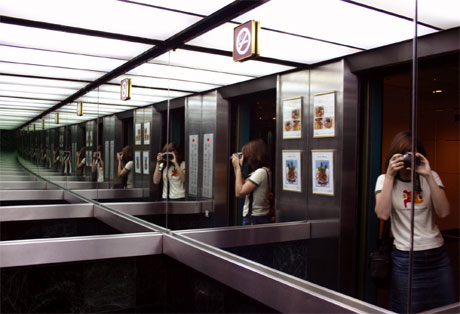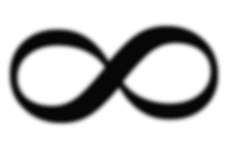A# infinityMystery has its own mysteries, and there are gods above gods. We have ours, they have theirs. That is what's known as infinity.
– Jean Cocteau (1889-1963), French author and filmmaker

Infinity is a concept that has always fascinated philosophers and theologians, linked as it is to the notions of unending distance or space, eternity, and God, but that was avoided or met with open hostility throughout most of the history of mathematics. Only within the past century or so have mathematicians dealt with it head on and accepted infinity as a number – albeit the strangest one we know.

An early glimpse of the perils of the infinite came to Zeno of Elae through his paradoxes, the best known of which pits Achilles in a race against a tortoise. Confident of victory, Achilles gives the tortoise a head start. But then how can he ever overtake the sluggish reptile? asks Zeno. First he must catch up to the point where it began, by which time the tortoise will have moved on. When he makes up the new distance that separated them, he finds his adversary has advanced again. And so it goes on, indefinitely. No matter how many times Achilles reaches the point where his competitor was, the tortoise has progressed a bit further. So perplexed was Zeno by this problem that he decided not only was it best to avoid thinking about the infinite but also that motion was impossible! A similar shock lay in store for Pythagoras and his followers who were convinced that everything in the universe could ultimately be understood in terms of whole numbers (even common fractions being just one whole number divided by another). The square root of 2 – the length of the diagonal of a right-angled triangle whose shorter sides are both one unit long – refused to fit into this neat cosmic scheme. It was an irrational number, inexpressible as the ratio of two integers. Put another way, its decimal expansion goes on forever without ever settling into a recurring pattern.These two examples highlight the basic problem in coming to grips with infinity. Our imaginations can cope with something that hasn't yet reached an end: we can always picture taking another step, adding one more to a total, or visualizing another term in a long series. But infinity, taken as a whole, boggles the mind. For mathematicians this was a particularly serious problem because mathematics deals with precise quantities and meticulously well-defined concepts. How could they work with things that clearly existed and went on indefinitely – a number like √2 or a curve that approached a line ever more closely – while avoiding a confrontation with infinity itself? Aristotle provided the key by arguing that there were two kinds of infinity. Actual infinity, or completed infinity, which he believed could not exist, is endlessness fully realized at some point in time. Potential infinity, which Aristotle insisted was manifest in nature – for example, in the unending cycle of the seasons or the indefinite divisibility of a piece of gold – is infinitude spread over unlimited time. This fundamental distinction persisted in mathematics for more than 2,000 years. In 1831 no less a figure than Karl Gauss expressed his "horror of the actual infinitude," saying:

I protest against the use of infinite magnitude as something completed, which is never permissible in mathematics. Infinity is merely a way of speaking, the true meaning being a limit which certain ratios approach indefinitely close, while others are permitted to increase without restriction.

By confining their attention to potential infinity, mathematicians were able to address and develop crucial concepts such as those of infinite series, limit, and infinitesimals, and so arrive at the calculus, without having to grant that infinity itself was a mathematical object. Yet as early as the Middle Ages certain paradoxes and puzzles arose, which suggested that actual infinity was not an issue to be easily dismissed. These puzzles stem from the principle that it is possible to pair off, or put in one-to-one correspondence, all the members of one collection of objects with all those of another of equal size. Applied to indefinitely large collections, however, this principle seemed to flout a commonsense idea first expressed by Euclid: the whole is always greater than any of its parts. For instance, it appeared possible to pair off all the positive integers with only those that are even: 1 with 2, 2 with 4, 3 with 6, and so on, despite the fact that positive integers also include odd numbers. Galileo, in considering such a problem, was the first to show a more enlightened attitude toward the infinite when he proposed that "infinity should obey a different arithmetic than finite numbers." Much later, David Hilbert offered a striking illustration of how weird the arithmetic of the endless can get.

Imagine, said Hilbert, a hotel with an infinite number of rooms. In the usual kind of hotel, with finite accommodation, no more guests can be squeezed in once all the rooms are full. But "Hilbert's Grand Hotel" is dramatically different. If the guest occupying room 1 moves to room 2, the occupant of room 2 moves to room 3, and so on, all the way down the line, a newcomer can be placed in room 1. In fact, space can be made for an infinite number of new clients by moving the occupants of rooms 1, 2, 3, etc, to rooms 2, 4, 6, etc, thus freeing up all the odd-numbered rooms. Even if an infinite number of coaches were to arrive each carrying an infinite number of passengers, no one would have to be turned away: first the odd-numbered rooms would be emptied as above, then the first coach's load would be put in rooms 3n for n = 1, 2, 3, ..., the second coach's load in rooms 5n for n = 1, 2, ..., and so on; in general, the people aboard coach number i would empty into rooms pn where p is the (i+1)th prime number.

Such is the looking-glass world that opens up once the reality of sets of numbers with infinitely many elements is accepted. That was a crucial issue facing mathematicians in the late nineteenth century: Were they prepared to embrace actual infinity as a number? Most were still aligned with Aristotle and Gauss in opposing the idea. But a few, including Richard Dedekind and, above all, Georg Cantor, realized that the time had come to put the concept of infinite sets on a firm logical foundation.

Cantor accepted that the well-known pairing-off principle, used to determine if two finite sets are equal, is just as applicable to infinite sets. It followed that there really are just as many even positive integers as there are positive integers altogether. This was no paradox, he realized, but the defining property of infinite sets: the whole is no bigger than some of its parts. He went on to show that the set of all positive integers, 1, 2, 3, ..., contains precisely as many members – that is, has the same cardinal number or cardinality – as the set of all rational numbers (numbers that can be written in the form p/q, where p and q are integers). He called this infinite cardinal number aleph-null, "aleph" being the first letter of the Hebrew alphabet. He then demonstrated, using what has become known as Cantor's theorem, that there is a hierarchy of infinities of which aleph-null is the smallest. Essentially, he proved that the cardinal number of all the subsets – the different ways of arranging the elements – of a set of size aleph-null is a bigger form of infinity, which he called aleph-one. Similarly, the cardinality of the set of subsets of aleph-one is a still bigger infinity, known as aleph-two. And so on, indefinitely, leading to an infinite number of different infinities.

Cantor believed that aleph-one was identical with the total number of mathematical points on a line, which, astonishingly, he found was the same as the number of points on a plane or in any higher n-dimensional space. This infinity of spatial points, known as the power of the continuum, c, is the set of all real numbers (all rational numbers plus all irrational numbers). Cantor's continuum hypothesis asserts that c = aleph-one, which is equivalent to saying that there is no infinite set with a cardinality between that of the integers and the reals. Yet, despite much effort, Cantor was never able to prove or disprove his continuum hypothesis. We now know why – and it strikes to the very foundations of mathematics.

In the 1930s, Kurt Gödel showed that it is impossible to disprove the continuum hypothesis from the standard axioms of set theory. Three decades later, Paul Cohen showed that it cannot be proven from those same axioms either. Such a situation had been on the cards ever since the emergence of Gödel's incompleteness theorem. But the independence of the continuum hypothesis was still unsettling because it was the first concrete example of an important question that provably could not be decided either way from the universally-accepted system of axioms on which most of mathematics is built.

Currently, the preference among mathematicians is to regard the Continuum Hypothesis as being false, simply because of the usefulness of the results that can be derived this way. As for the nature of the various types of infinities and the very existence of infinite sets, these depend crucially on what number theory is being used. Different axioms and rules lead to different answers to the question what lies beyond all the integers? This can make it difficult or even meaningless to compare the various types of infinities that arise and to determine their relative size, although within any given number system the infinities can usually be put into a clear order. Certain extended number systems, such as the surreal numbers, incorporate both the ordinary (finite) numbers and a diversity of infinite numbers. However, whatever number system is chosen, there will inevitably be inaccessible infinities – infinities that are larger than any of those the system is capable of producing.

### References

1. Lavine, S. Understanding the Infinite. Cambridge, Mass.: Harvard University Press, 1994.
2. Maor, E. To Infinity and Beyond: A Cultural History of the Infinite. Boston, Mass.: Birkhäuser, 1987.
3. Rucker, Rudy. Infinity and the Mind. Princeton, NJ: Princeton University Press, 1995.